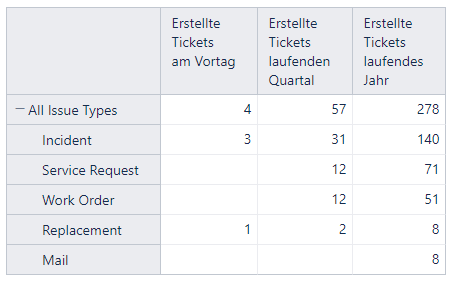# Average number of created issues per day

With this formula I get the total number of issues created in current year:

Sum(
Filter(
Descendants([Issue].CurrentHierarchyMember,[Issue].[Issue]),
(DateInPeriod([Issue].CurrentHierarchyMember.get(‘Created at’), [Time].[Year].CurrentDateMember)
)
),
[Measures].[Issues created]
)

I would like to get the average of issues that have been created per day in current year. In this report where I count number of items created yesterday, the current quarter and the current year:Any idea how to get this?

Hi @AntonBDR,

there is a similar post here that has a solution: Average of issues created by week

Regards,

Fabian

Hi @AntonBDR ,

To return the number of issues created in the current year, I recommend a more straightforward formula without iterating through issues with Descendants(). See the suggested formula below:

``````([Measures].[Issues created],[Time].[Year].CurrentDateMember)
``````

That will also improve the report performance.

Next, to return the average number of issues created per day, use the DateDiffDays() function to calculate the number of days passed since the start of the current year and now. Then you can divide the number of issues created by the number of days passed. See the suggested formula below:

``````CASE WHEN
val(([Measures].[Issues created],[Time].[Year].CurrentDateMember)) > 0
THEN
val(([Measures].[Issues created],[Time].[Year].CurrentDateMember)
/
DateDiffDays(
[Time].[Year].CurrentDateMember.StartDate,
Now()
))
END
``````

Visit the eazyBI documentation page for more details on functions used in the report - MDX Function Reference.

Best,
Roberts // support@eazybi.com

1 Like

Thanks a lot @roberts.cacus that works pretty good.
Would this count the total number of days in the time period? or the number of working days? If there are no issues created in a day would it be counted?

Now I would like to do the same calculating the avg of the last 180 days. How would the formula look like?

Hi @AntonBDR ,

The suggested calculation looks for all days, even when there were no issues created. To consider only working days, replace the `DateDiffDays()` function in the formula with `DateDiffWorkdays()`.

For the last 180 days, I recommend defining a Time dimension calculated member aggregating the last 180 “Day” members. See the suggested formula below:

``````-- Last 180 days
Aggregate(
[Time].[Day].DateMembersBetween('180 days ago', 'today')
)
``````

Then you can use this calculated Time dimension member in a formula similar to the one below:

``````CASE WHEN
val(([Measures].[Issues created],[Time].[Last 180 days])) > 0
THEN
val(([Measures].[Issues created],[Time].[Last 180 days])
/
180)
END
``````

Please look at the eazyBI documentation page for more information on defining calculated measures and members - ​https://docs.eazybi.com/eazybijira/analyze-and-visualize/calculated-measures-and-members.

Best,
Roberts // support@eazybi.com

1 Like

how could I count the number of days between the 1st day of the Quarter and Today ?

Something like this:

Aggregate(
[Time].[Day].DateMembersBetween(## First day of the Quarter ##, ‘today’)
)

Hi @AntonBDR,

It depends on which quarter you want to consider. Suppose it is the current quarter. Then the formula to count the number of days from the start of the quarter till today could look similar to the one below:

``````DateDiffDays(
[Time].[Quarter].CurrentDateMember.StartDate,
'today'
)
``````

Best,
Roberts // support@eazybi.com

1 Like